append 订阅

StringBuffer

$(selector).append(content) 书 名 《Java 2 入门经典（JDK 5)》 释 义 在被选元素的结尾（仍然在内部）插入指定内容 外文名 append 作 者 Ivor Horton appendjquery 文档操作 - append() 方法 在每个 p 元素结尾插入内容：$("button").click(function(){ $("p").append(" Hello world!"); });append() 方法在被选元素的结尾（仍然在内部）插入指定内容。提示：如需在被选元素的开头插入内容，请使用prepend()方法。提示：append() 和appendTo()方法执行的任务相同。不同之处在于：内容的位置和选择器。$(selector).append(content)   使用函数在指定元素的结尾插入内容。$(selector).append(function(index,html))  收起全文精华内容 下载资源 问答 • ## np.append()函数用法 万次阅读 多人点赞 2019-06-28 23:21:49 函数np.append(arr, values, axis=None) 作用： 为原始array添加一些values 参数： arr:需要被添加values的数组 values:添加到数组arr中的值（array_like，类数组） axis:可选参数，如果axis没有给出，那么arr... 函数np.append(arr, values, axis=None) 作用： 为原始array添加一些values 参数： arr:需要被添加values的数组 values:添加到数组arr中的值（array_like，类数组） axis:可选参数，如果axis没有给出，那么arr，values都将先展平成一维数组。注：如果axis被指定了，那么arr和values需要有相同的shape，否则报错：ValueError: arrays must have same number of dimensions 补充对axis的理解 axis的最大值为数组arr的维数-1，如arr维数等于1，axis最大值为0；arr维数等于2，axis最大值为1，以此类推。 当arr的维数为2(理解为单通道图)，axis=0表示沿着行方向添加values；axis=1表示沿着列方向添加values 当arr的维数为3(理解为多通道图)，axis=0，axis=1时同上；axis=2表示沿着深度方向添加values 返回： 添加了values的新数组 e.g. 1. 不考虑axis arr，values都将先展平成一维数组,然后沿着axis=0的方向在arr后添加values import numpy as np a=[1,2,3] b=[4,5] c=[[6,7],[8,9]] print(np.append(a,b)) print(np.append(a,c)) 输出结果如下： [1 2 3 4 5] [1 2 3 6 7 8 9]  2. 考虑axis arr,values的shape相同 import numpy as np a=[1,2,3] b=[4,5] c=[[6,7],[8,9]] d=[[10,11],[12,13]] print('在一维数组a后添加values,结果如下：{}'.format(np.append(a,b,axis=0))) print('沿二维数组c的行方向添加values结果如下：'.format(np.append(c,d,axis=0))) print('沿二维数组c的列方向添加values结果如下：'.format(np.append(c,d,axis=1))) print('使用了axis，若arr和values的形状不同，则报错：'.format(np.append(a,c,axis=0))) 结果如下： 在一维数组a后添加values,结果如下： [1 2 3 4 5] 沿二维数组c的行方向添加values结果如下： [[ 6 7] [ 8 9] [10 11] [12 13]] 沿二维数组c的列方向添加values结果如下： [[ 6 7 10 11] [ 8 9 12 13]] 3. 考虑axis，如果arr和values的形状不同，则报错： import numpy as np a=[1,2,3] c=[[6,7],[8,9]] print(np.append(a,c,axis=0))  输出结果如下： Traceback (most recent call last): File "F:\eclipse-workspace\test\t1.py", line 4, in <module> print(np.append(a,c,axis=0)) File "E:\anaconda\anzhuang\lib\site-packages\numpy\lib\function_base.py", line 4694, in append return concatenate((arr, values), axis=axis) ValueError: all the input arrays must have same number of dimensions PS: 偷偷给自己打波小广告，扫码关注微信公众号获取深度学习数据集 我们不生产数据，我们只是数据的搬运工展开全文numpy python • ## python中append函数的用法 万次阅读 多人点赞 2018-11-12 11:08:47 append函数会在数组后加上相应的元素 例： a=[1,2,3] a.append(5) 此时，运行结果为 [1, 2, 3, 5] a=[1,2,3] a.append() 此时，运行结果为 [1, 2, 3, ] 结果不再为一个数组，而是list 用append生成... append函数会在数组后加上相应的元素 例： a=[1,2,3] a.append(5) 此时，运行结果为 [1, 2, 3, 5] a=[1,2,3] a.append() 此时，运行结果为 [1, 2, 3, ] 结果不再为一个数组，而是list 用append生成多维数组： import numpy as np a=[] for i in range(5): a.append([]) for j in range(5): a[i].append(i) 结果如下： [[0, 0, 0, 0, 0], [1, 1, 1, 1, 1], [2, 2, 2, 2, 2], [3, 3, 3, 3, 3], [4, 4, 4, 4, 4]] 矩阵转置函数transpose方法： a=np.transpose(a) 结果如下：  ([[0, 1, 2, 3, 4], [0, 1, 2, 3, 4], [0, 1, 2, 3, 4], [0, 1, 2, 3, 4], [0, 1, 2, 3, 4]]) 数组合并操作： h=np.arange(-2,2,1) h.shape k1=np.c_[h,h] #横向合并函数1 np.c_，将数组转化为列向量 k2=np.hstack((h,h)) #横向合并函数2 np.hstack，将数组作为横向量 print("k1="+str(k1)) print("k2="+str(k2)) l1=np.r_[[h],[h]] #纵向合并函数np.r_ l2=np.vstack((h,h)) #纵向合并函数np.vstack print("l1="+str(l1)) print("l2="+str(l2)) 结果如下： k1=[[-2 -2] [-1 -1] [ 0 0] [ 1 1]] k2=[-2 -1 0 1 -2 -1 0 1] l1=[[-2 -1 0 1] [-2 -1 0 1]] l2=[[-2 -1 0 1] [-2 -1 0 1]] 展开全文python • ## pandas的DataFrame的append方法详细介绍 万次阅读 多人点赞 2018-12-11 22:18:06 官方文档介绍链接：append方法介绍 DataFrame.append(other, ignore_index=False, verify_integrity=False, sort=None) 功能说明：向dataframe对象中添加新的行，如果添加的列名不在dataframe对象中，将会被当作新的... 官方文档介绍链接：append方法介绍 DataFrame.append(other, ignore_index=False, verify_integrity=False, sort=None) 功能说明：向dataframe对象中添加新的行，如果添加的列名不在dataframe对象中，将会被当作新的列进行添加 other：DataFrame、series、dict、list这样的数据结构 ignore_index：默认值为False，如果为True则不使用index标签 verify_integrity ：默认值为False，如果为True当创建相同的index时会抛出ValueError的异常 sort：boolean，默认是None，该属性在pandas的0.23.0的版本才存在。 append添加字典  import pandas as pd data = pd.DataFrame() a = {"x":1,"y":2} data = data.append(a,ignore_index=True) print(data)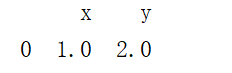append添加series 如果不添加ignore_index=True，会报错提示TypeError: Can only append a Series if ignore_index=True or if the Series has a name，如果不添加ignore_index=True，也可以改成以下代码  import pandas as pd data = pd.DataFrame() series = pd.Series({"x":1,"y":2},name="a") data = data.append(series) print(data)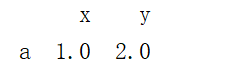注意：当dataframe使用append方法添加series的时候，必须要设置name，设置name名称将会作为index的name。 append添加list  data = pd.DataFrame() a = [1,2,3] data = data.append(a) print(data)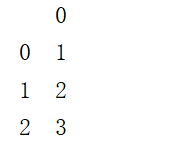如果list是一维的，则是以列的形式来进行添加，如果list是二维的则是以行的形式进行添加的，如果是三维的则只添加一个值  data = pd.DataFrame() a = [[[1,2,3]]] data = data.append(a) print(data)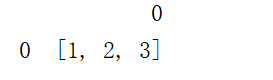注意：在多次使用append方法追加数据的时候，可能会出现相同的index  data = pd.DataFrame() a = [[1,2,3],[4,5,6]] data = data.append(a) a = [[7,8,9],[10,11,12]] data = data.append(a) print(data)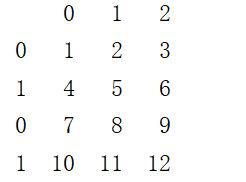如果想要添加的index不出现重复的情况，可以通过设置ignore_index=True来避免  data = pd.DataFrame() a = [[1,2,3],[4,5,6]] data = data.append(a,ignore_index=True) a = [[7,8,9],[10,11,12]] data = data.append(a,ignore_index=True) print(data)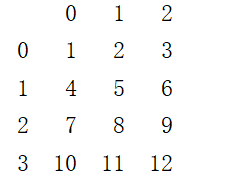展开全文• ## append用法 万次阅读 多人点赞 2019-05-27 20:46:38 #append() a = [] a.append(['A','B','C'])#给列表添加列表 a.append('a')#给列表添加字符串，字符串必须引号 a.append(5)#给列表添加整数，浮点都不用加引号，加引号就转换成字符串了 print(a) 运行结果为 [['A',... #append() a = [] a.append(['A','B','C'])#给列表添加列表 a.append('a')#给列表添加字符串，字符串必须引号 a.append(5)#给列表添加整数，浮点都不用加引号，加引号就转换成字符串了 print(a) 运行结果为 [['A', 'B', 'C'], 'a', 5]  展开全文• 一、append命令 1.append 简单例子 redis>EXISTS mykey//是否存在mykey (integer) 0 redis>APPEND mykey "Hello" (integer) 5 redis>APPEND mykey "... • func append(slice []Type, elems ...Type) []Type The append built-in function appends elements to the end of a slice. If it has sufficient capacity, the destination is resliced to accommodate t...golang • ## numpy append 千次阅读 2019-04-28 18:30:52 import numpy as np a = np.array([[1,2,3]...print(np.append(a,[[5,5,5],[7,8,9]],axis=1)) [[1 2 3 5 5 5] [4 5 6 7 8 9]] 在a每一层的每一层后面添加 print(np.append(a,[[5,5,5],[7,8,9]],axis=0)) [[1 2... • ## 数据append 千次阅读 2018-07-19 17:20:29 循环将多个txt文件中的数据append到已存在的csv中，换行，不删除已有数据 读入的d0a32932-d418-4290-9fb3-02318fb37039.txt数据格式： 61 11 163 103 126 42 247 125 318 217 492 381 422 22 502 94 输出的csv数据...Python csv e • Append 是后面的内容附给前面； append to 是其前面的内容付给后面 和after 的区别 • ## golang append数组 千次阅读 2020-07-05 15:28:22 golang切片append一个数组：list = append(append, anotherList...) mylist := make([]int, 0, 10) mylist1 := []int{1, 2, 3} mylist = append(mylist, mylist1...) //加上'...'就可以追加一个数组， 否则只能...golang • ## c++ append用法 万次阅读 多人点赞 2017-11-16 15:33:47 append函数是向string的后面追加字符或字符串。 1).向string的后面加C-string string s = “hello “; const char *c = “out here “; s.append(c); // 把c类型字符串s连接到当前字符串结尾 s = “hello out...c++ • 总结：关于Nologging与append测试的一些总结，通过上面的SQL语句查看可以得出在大量数据插入过程的语句中加入/*+append*/的这个SQL语句产生的REDO日志明显示是会少同时时间节约了很多，当然这样可能会影响备份因此... • ## sys.path.append()用法 万次阅读 2017-12-27 10:28:56 该路径已经添加到系统的环境变量了，当我们要添加自己的搜索目录时，可以通过列表的append()方法；对于模块和自己写的脚本不在同一个目录下，在脚本开头加sys.path.append(‘xxx’)：import sys sys.path.append(’... • HTML中$('#scid').append("123"); 追加清空是用空的; remove() 不是清空这个元素，而是把它删除掉，删都删掉了就不能看到勒呗 - - 清空用 empty()！！！HTML js
• python中的append()函数是在列表末尾添加新的对象，且将添加的对象最为一个整体。与append相对应的是extend函数。网上有很多对这两个函数的区别讲解，但我觉得都讲的不是很清楚，记忆不深刻。这样解释清楚且容易记住...
• python append（）函数 一、list中append()与extend()用法 1. 列表可包含任何数据类型的元素，单个列表中的元素无须全为同一类型。  2. append() 方法向列表的尾部添加一个新的元素。只接受一个参数。 3. ...
• 今天就来简单介绍一下NumPy 和 Python中append()函数的特点，以及append()的特性是否适合用于大数据的处理。 NumPy 和 Python中append()函数的特点 首先我们来复习一下Python中的append()用法，作为Python中最为常见...NumPy Python
• ## java append方法

万次阅读 多人点赞 2018-02-09 16:35:30
java append方法StringBuffer buf = new StringBuffer("Hard ")；String aString = "Waxworks"；buf.append(aString,3,4);原文说明：这个操作将aString的从索引位置3开始的由四个字符组成的子串...
• ## Python的常用函数：append()

万次阅读 多人点赞 2019-07-08 15:19:48
Python的常用函数：append() 1. 描述 append()函数用于在列表末尾添加新的对象。 2. 语法 list.append(obj) 其中： list:列表对象； obj:添加到列表末尾的对象。 注意：append()函数无返回值，但是会修改原本的列表...Python
• 今天突发奇想。。 给list.append()赋值，后发现返回值为none 理解为： b = a.append(3) 因为a.append(3) 是一个none type ，返回值为None，所以 对b赋值，就为None ...
• append()函数描述：在列表ls最后(末尾)添加一个元素object语法：ls.append(object) -> None 无返回值例：a=[1,2,3]a.append(5)此时，运行结果为 [1, 2, 3, 5]a=[1,2,3]a.append()此时，运行结果为 [1, 2, 3, ...
• ## append() 方法

万次阅读 2019-09-08 22:45:28
ls.append(1) return ls a=func() b=func() print(a,b) 运行结果 2.append() 方法用于在列表末尾添加新的对象。 def func(ls=): ls.append(1) return ls a=func() b=func() print(a,b) 3...
• 1.append 不修改切片内容，所以一般写法 s1 = append（s1,xxx） go append 切片增加元素的时候并不会修改切片的内容，但是如果容量足够的情况下，会修改切片指向地址的内容。 2.append 如果不足以容纳附加的新内容...go
• Redis 之 append   如果key 不存在, 调用 append 命令 append key value 会新建一个key, 并将value 设置为key的值,类似于set         如果key 存在, 调用 append 命令 append key value 会新建一个key...
• ## abap append 用法

千次阅读 2019-09-22 09:55:15
【转自http://blog.chinaunix.net/uid-7982817-id-91999.html】Append用法总结2008-11-14 11:42:19 分类： ...APPEND { wa | {INITIAL LINE} | {LINES OF jtab [FROM idx1] [TO idx2]} }...
• 今天就来简单介绍一下NumPy 和 Python中append()函数的特点，以及append()的特性是否适合用于大数据的处理。 NumPy 和 Python中append()函数的特点 首先我们来复习一下Python中的append()用法，作为Python中最为常见...
• append方法的作用是在一个StringBuffer对象后面追加字符串。 例如 StringBuffer s = new StringBuffer(“Hello”); s.append(“World”); 则s的内容是HelloWorld public String printStr() { StringBuffer sbu=new ...
• python中的append()函数是在列表末尾添加新的对象，且将添加的对象最为一个整体。与append相对应的是extend函数。网上有很多对这两个函数的区别讲解，但我觉得都讲的不是很清楚，记忆不深刻。这样解释清楚且容易记住...
• ## golang的append

千次阅读 2018-08-31 22:44:07
package main import "fmt" func main(){ a:=make([]int,1) fmt.Println(a) a=append(a,2)//len=2,cap=2 ... a=append(a,3)//len=3,cap=4 b:=append(a,4)//len=4,cap=4 a=append(a,3)//len=4,c...
• ## append 例子

千次阅读 2013-11-17 09:44:36
append方法的作用是在一个StringBuffer对象后面追加字符串。   例如 StringBuffer s = new StringBuffer("Hello"); s.append("World"); 则s的内容是HelloWorld...